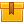# Controller Tuning (ACTAV)

## Prerequisites

This lesson is designed for participants familiar with the basics of instrument control including the proportional, integral, and derivative control modes.

## Description

This lesson explains tuning methods and principles behind the three methods of tuning a controller: ultimate, reaction curve, and trial and error. The lesson presents the characteristics of a properly timed process response and relates these to proportional, integral, and derivative control actions. In addition, step-by-step procedures for tuning controllers using each method are demonstrated.

## Objectives

Define controller tuning and its purpose as used in a process control system. Define a process response. Explain the significance of a 1/4 decay reaction curve. Perform the preliminary steps for tuning a controller. Stabilize a process on manual control before using the ultimate method. Obtain the value of and calculate the proper setting for a proportional band or gain. Find the value of the ultimate period. Calculate the proper settings for PI, PD, and PID controllers. Verify adjustments. Obtain and interpret a process reaction curve.

Calculate the process gain, dead time, time constant, and controller settings using the reaction curve method. Interpret a process response to determine the proper setting. Using the trial and error method, tune the proportional mode.

## ContentThis lesson explains tuning methods and principles behind the three
methods of tuning a controller: ultimate, reaction curve, and trial and
error. The lesson presents the characteristics of a properly timed
process response and relates these to proportional, integral, and
derivative control actions. In addition, step-by-step procedures for
tuning controllers using each method are demonstrated.
•Controller Tuning

0
0 Reviews